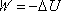W = minus delta U

Work = the negative of the change in potential energy

This represents the defining equation for potential energy.

In the case of an object that moves from point A to point B under the influence of a conservative force, the work done, calculated by means of the definition (the force-times-distance method) always turns out to be the same value, no matter what path the object takes in going from A to B. As such, one can assign a value with units of energy to each point in space (called the potential energy at that point in space) such that the work done by the conservative force under consideration is equal to the negative of the following quantity: the potential energy at point B minus the potential energy at point A.